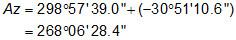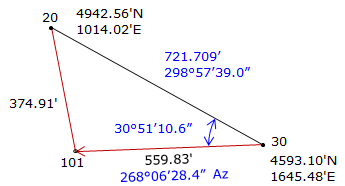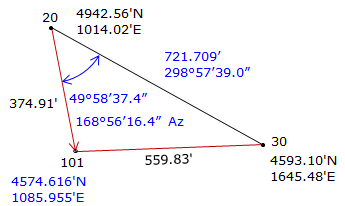### Article Index

Two different intersection types are solved using triangle- and arc-based methods to demonstrate the different computation process. Additional decimal places will be carried in computations to minimize rounding errors.

### 1. Distance-distanceGiven the information on the diagram, determine the coordinates of point 101. Figure E-1 Distance-distance example

Step (1) For both methods is to inverse along the base line 30-20#### a. Triangle-based method

 Step (2) Compute angle at 30 by Law of Cosines.Step (3) Compute direction from 30 to 101.Step (4) Perform a forward computation from 30 to 101.Math Check: Compute coordinates from 20.

 Step (1) Compute angle at 20 by Law of Sines.Step (2) Compute direction from 20 to 101.Step (3) Perform a forward computation from 20 to 101.Both coordinates check.#### b. Arc-based method

Step (2) Set up and solve Equations D-6 through D-9.Step (3) Use Equations D-10 and D-11 to compute the two intersection pointsStep (4) Of the two, select the appropriate intersection point.

Point 101 is located southwest of the base line.

 Point North East From base line 1011 5077.015 1363.991 north east 1012 4574.617 1085.956 south west

The correct intersection point is 1012: (4754.617 ft N, 1085.956 ft E), same as the triangle-based solution.

### 2. Direction-distance

Figure xx-2 is a diagram of a base line and an intersection point.Figure E-2 Direction-distance example

Coordinates of the base line end points are:

 Point North (ft) East (ft) J 1419.51 3511.69 K 2056.64 2341.36

The azimuth from point J to point T is 329°46'45"; the distance from point K to point T is 738.15 ft.

What are the coordinates of point T?

Step (1) Inverse the base line from point K to point J#### a. Triangle-based method

To get point T's coordinates, we'll perform a forward computation from K.

Step (2) Compute the angle at JStep (3) Use Law of Sines to compute angle at TRemember that we have to check the angle returned by the Law of Sines because it will always be <90°.

From the diagram, T is greater than 90° so it must be subtracted from 180°Step (4) Compute angle at K from the Angle ConditionStep (5) Compute azimuth from point K to point TStep (6) Forward compute from point KThe math check is to compute the coordinates from point J

Step (1) Compute distance from point J using Law of SinesStep (2) Forward compute from point J#### b. Arc-based method

Because the direction can intersect the arc at two points, we'll extend the diagram a bit to visualize the possibilities, Figure XX-XX.Figure E-3 Direction-distance arc example

Step (2) Set up and solve Equations D-12 through D-15.Step (3) Pick the correct distance and compute the coordinates

From Figure E-3, the correct distance dQ is the shorter one: 878.915,. Using that distance, perform a forward computationThe coordinates of point T are the same as those from the triangle-based method.